# Graphing Speed and Acceleration Graphing Speed Distance vs

• Slides: 14Graphing Speed and Acceleration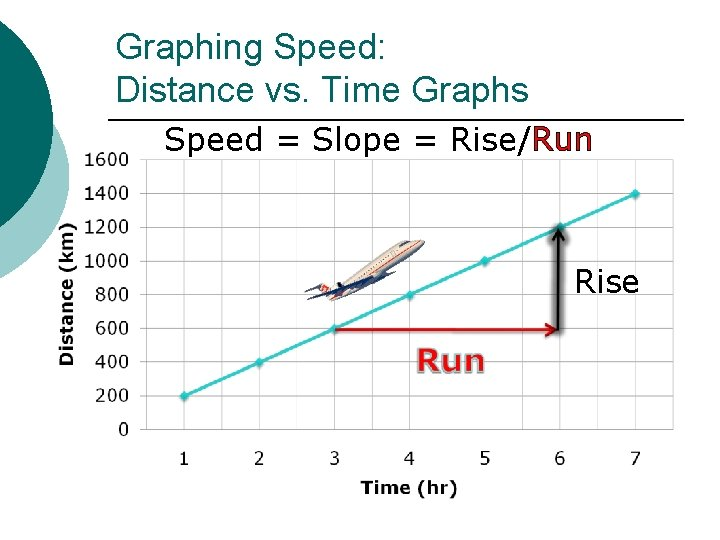Graphing Speed: Distance vs. Time Graphs Speed = Slope = Rise/Run Rise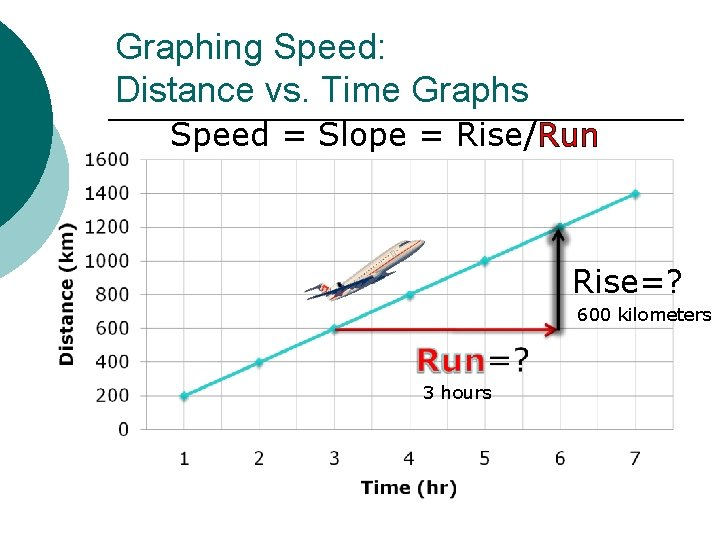Graphing Speed: Distance vs. Time Graphs Speed = Slope = Rise/Run Rise=? 600 kilometers 3 hours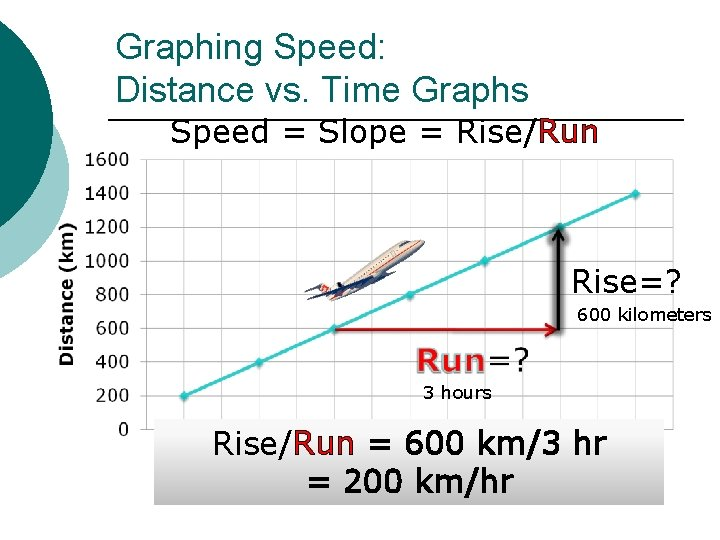Graphing Speed: Distance vs. Time Graphs Speed = Slope = Rise/Run Rise=? 600 kilometers 3 hours Rise/Run = 600 km/3 hr = 200 km/hr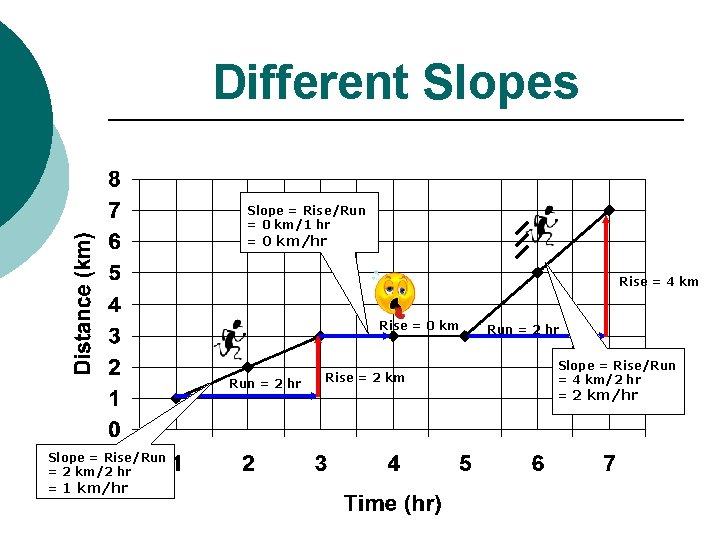Different Slopes Slope = Rise/Run = 0 km/1 hr = 0 km/hr Rise = 4 km Rise = 0 km Run = 2 hr Slope = Rise/Run = 2 km/2 hr = 1 km/hr Rise = 2 km Run = 2 hr Slope = Rise/Run = 4 km/2 hr = 2 km/hr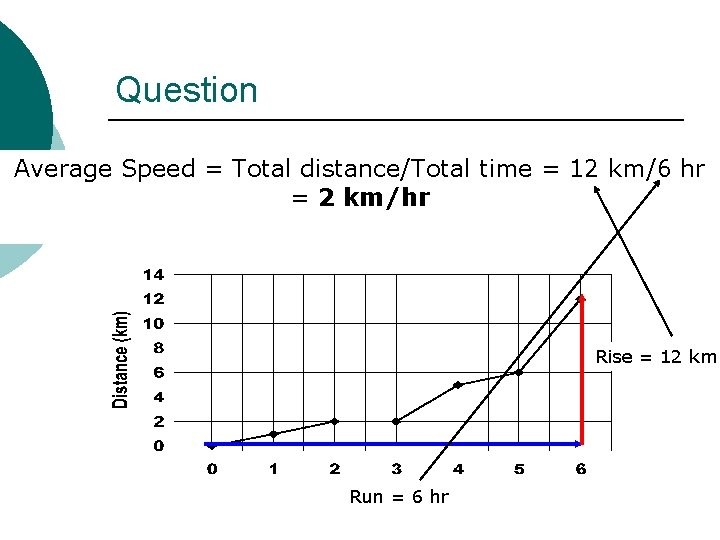Question Below= is a distance vs. time graph of km/6 hr Average ¡Speed Total distance/Total time = 12 my position =during a race. What was 2 km/hr my AVERAGE speed for the entire race? Rise = 12 km Run = 6 hr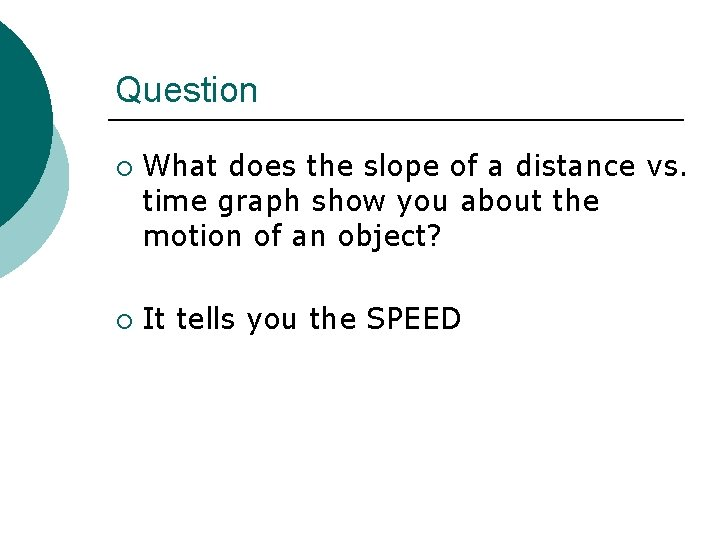Question ¡ ¡ What does the slope of a distance vs. time graph show you about the motion of an object? It tells you the SPEED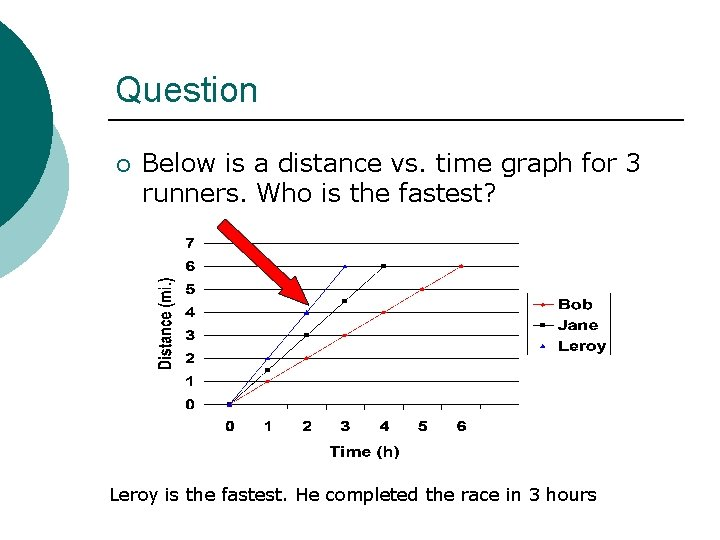Question ¡ Below is a distance vs. time graph for 3 runners. Who is the fastest? Leroy is the fastest. He completed the race in 3 hoursGraphing Acceleration ¡ Can use 2 kinds of graphs l l Speed vs. time Distance vs. timeGraphing Acceleration: Speed vs. Time Graphs 1) Speed is increasing with time = accelerating 2) Line is straight = acceleration is constant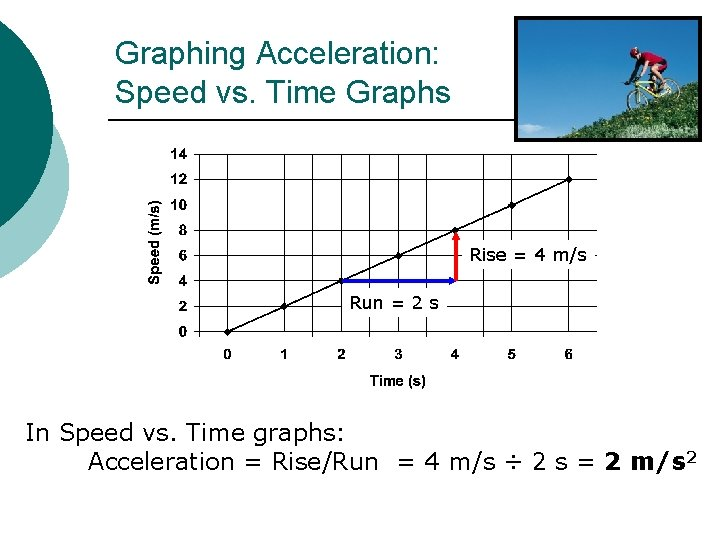Graphing Acceleration: Speed vs. Time Graphs Rise = 4 m/s Run = 2 s In Speed vs. Time graphs: Acceleration = Rise/Run = 4 m/s ÷ 2 s = 2 m/s 2Graphing Acceleration: Distance vs. Time Graphs 1) On Distance vs. Time graphs a curved line means the object is accelerating. 2) Curved line also means your speed is increasing. Remember slope = speed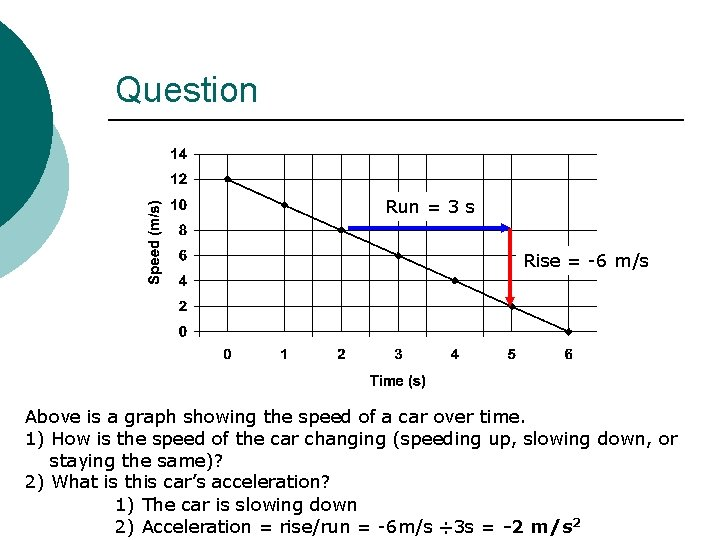Question Run = 3 s Rise = -6 m/s Above is a graph showing the speed of a car over time. 1) How is the speed of the car changing (speeding up, slowing down, or staying the same)? 2) What is this car’s acceleration? 1) The car is slowing down 2) Acceleration = rise/run = -6 m/s ÷ 3 s = -2 m/s 2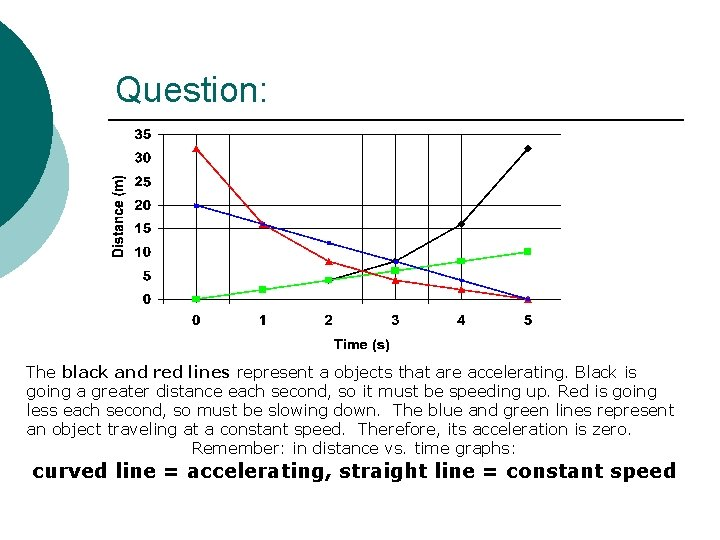Question: The black and red lines represent a objects that are accelerating. Black is going a greater distance each second, so it must be speeding up. Red is going less each second, so must be slowing down. The blue and green lines represent an object traveling at a constant speed. Therefore, its acceleration is zero. 1)Which line represents Remember: in distance vs. an timeobject graphs: that is curved line = accelerating, straight line = constant speed accelerating?